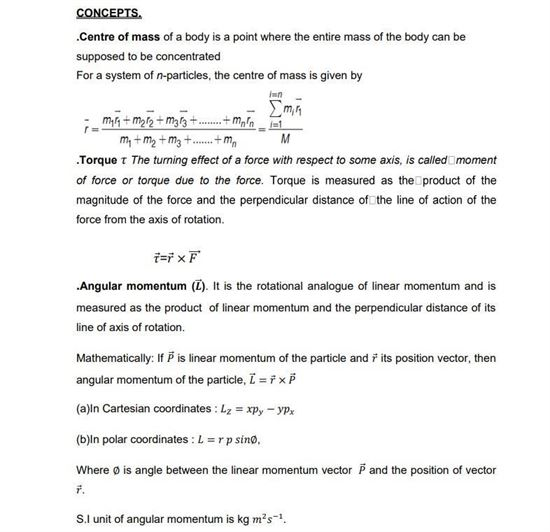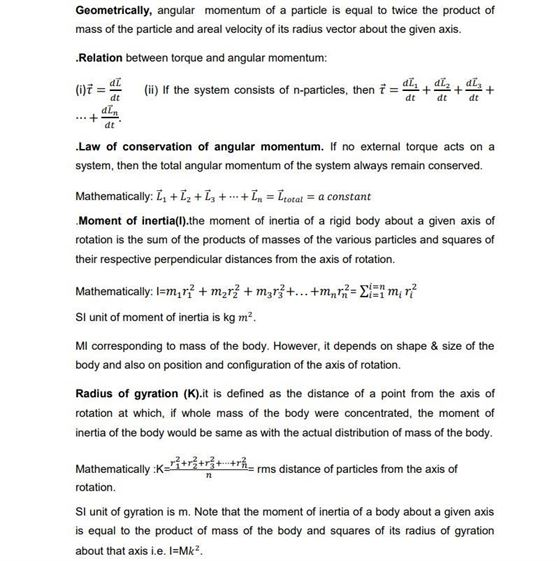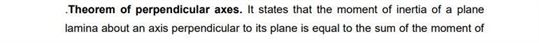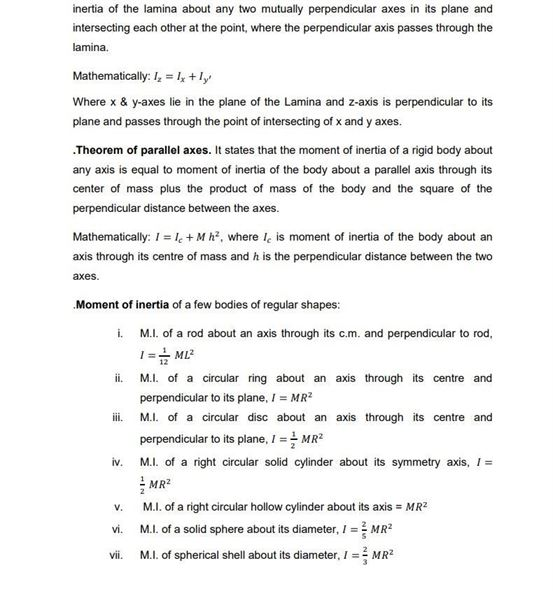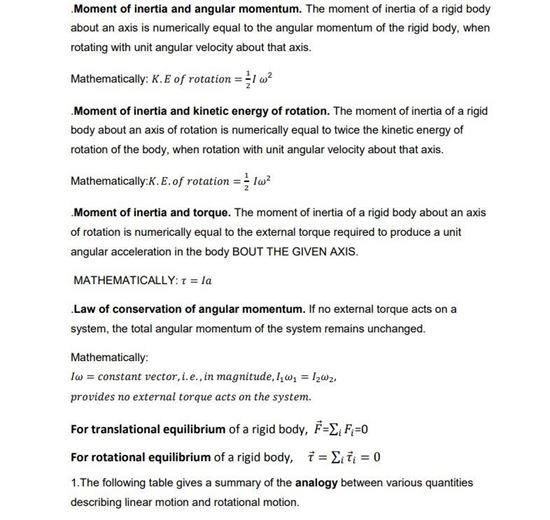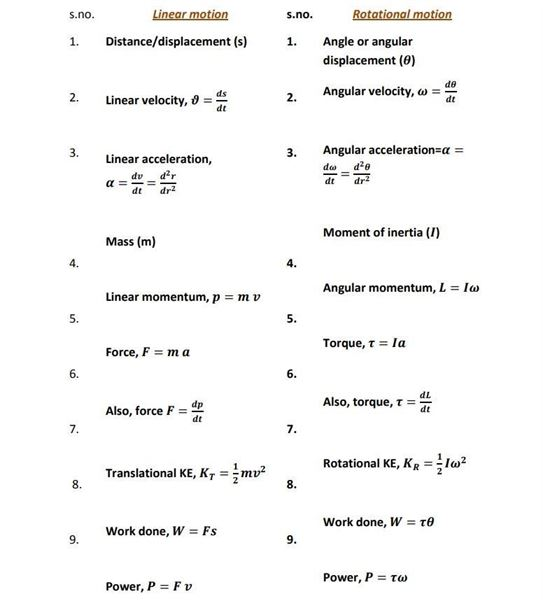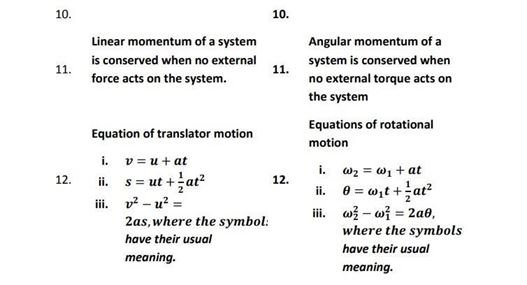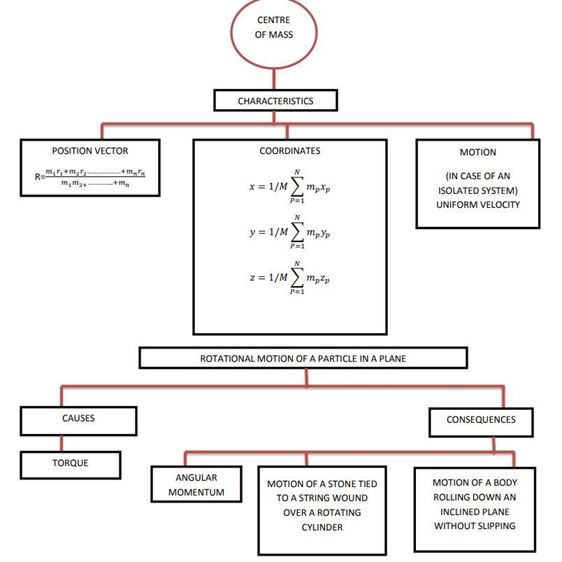# Physics Revision Notes for Class 11 Chapter 7 System of Particles and Rotational Motion

The class 11 Physics Revision Notes Chapter 7 System of Particles and Rotational Motion is one of the important chapters when it comes to understanding the basics of circular motion. We all are aware of the definition of rotational motion, the center of mass and center of gravity etc.

• If a body is pivoted at a point and the force is applied to the body at a suitable point, it rotates the body about the axis passing through the pivoted point. This is the turning effect of the force and the motion of the body is called the Rotational motion
• Center of Mass is the point in a body or system of bodies at which the whole mass may be considered as concentrated
• Center of Gravity is the point at which the entire weight of a body may be considered as concentrated so that if supported at this point the body would remain in equilibrium in any position

In this notes, students will get to know the vector product and cross product of vectors and even what is angular acceleration, torque, angular momentum, the moment of inertia, the radius of gyration. Preparing from this notes the important topics such as the theorem of the parallel axis and perpendicular axis will fetch a good score in exams.

Check out the physics revision notes for class 11 chapter 7 System of Particles and Rotational Motion here.